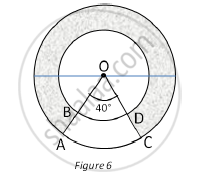# In Fig. 6, find the area of the shaded region, enclosed between two concentric circles of radii 7 cm and 14 cm where ∠AOC = 40°. - Mathematics

In Fig. 6, find the area of the shaded region, enclosed between two concentric circles of radii 7 cm and 14 cm where ∠AOC = 40°. (use pi =  22/7)#### Solution

Area of the region ABDC = Area of sector AOC – Area of sector BOD

=40^@/360^@xx22/7xx14xx14-40^@/360^@xx22/7xx7xx7

=1/9xx22xx14xx2-1/9xx22xx7xx1

=22/9xx(28-7)

=22/9xx21

=154/3

=51.33 cm2

Area of circular ring = 22/7xx14xx14-22/7xx7xx7

= 22x14x2-22x7x1

=22x(28-7)

=22x21

=462 cm2

∴ Required shaded region Area of circular ring Area of region ABDC

= 462 - 51.33

= 410.67 cm2

Thus, the area of shaded region is 410.67 cm2

Concept: Circumference of a Circle
Is there an error in this question or solution?